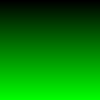# Write a PNG file using C and libpngThis C program creates the simple image on the right, and then writes it to a PNG file called `fruit.png`.

This program is based on an example I found using an internet search engine, which I then altered so that it worked. The documentation for libpng is a bit difficult to understand. I hope that the complete specification of the library is in there, but I couldn't find some things.

```#include <png.h>
#include <stdio.h>
#include <stdlib.h>
#include <stdint.h>

/* A coloured pixel. */

typedef struct
{
uint8_t red;
uint8_t green;
uint8_t blue;
}
pixel_t;

/* A picture. */

typedef struct
{
pixel_t *pixels;
size_t width;
size_t height;
}
bitmap_t;

/* Given "bitmap", this returns the pixel of bitmap at the point
("x", "y"). */

static pixel_t * pixel_at (bitmap_t * bitmap, int x, int y)
{
return bitmap->pixels + bitmap->width * y + x;
}

/* Write "bitmap" to a PNG file specified by "path"; returns 0 on
success, non-zero on error. */

static int save_png_to_file (bitmap_t *bitmap, const char *path)
{
FILE * fp;
png_structp png_ptr = NULL;
png_infop info_ptr = NULL;
size_t x, y;
png_byte ** row_pointers = NULL;
/* "status" contains the return value of this function. At first
it is set to a value which means 'failure'. When the routine
has finished its work, it is set to a value which means
'success'. */
int status = -1;
/* The following number is set by trial and error only. I cannot
see where it it is documented in the libpng manual.
*/
int pixel_size = 3;
int depth = 8;

fp = fopen (path, "wb");
if (! fp) {
goto fopen_failed;
}

png_ptr = png_create_write_struct (PNG_LIBPNG_VER_STRING, NULL, NULL, NULL);
if (png_ptr == NULL) {
goto png_create_write_struct_failed;
}

info_ptr = png_create_info_struct (png_ptr);
if (info_ptr == NULL) {
goto png_create_info_struct_failed;
}

/* Set up error handling. */

if (setjmp (png_jmpbuf (png_ptr))) {
goto png_failure;
}

/* Set image attributes. */

png_set_IHDR (png_ptr,
info_ptr,
bitmap->width,
bitmap->height,
depth,
PNG_COLOR_TYPE_RGB,
PNG_INTERLACE_NONE,
PNG_COMPRESSION_TYPE_DEFAULT,
PNG_FILTER_TYPE_DEFAULT);

/* Initialize rows of PNG. */

row_pointers = png_malloc (png_ptr, bitmap->height * sizeof (png_byte *));
for (y = 0; y < bitmap->height; y++) {
png_byte *row =
png_malloc (png_ptr, sizeof (uint8_t) * bitmap->width * pixel_size);
row_pointers[y] = row;
for (x = 0; x < bitmap->width; x++) {
pixel_t * pixel = pixel_at (bitmap, x, y);
*row++ = pixel->red;
*row++ = pixel->green;
*row++ = pixel->blue;
}
}

/* Write the image data to "fp". */

png_init_io (png_ptr, fp);
png_set_rows (png_ptr, info_ptr, row_pointers);
png_write_png (png_ptr, info_ptr, PNG_TRANSFORM_IDENTITY, NULL);

/* The routine has successfully written the file, so we set
"status" to a value which indicates success. */

status = 0;

for (y = 0; y < bitmap->height; y++) {
png_free (png_ptr, row_pointers[y]);
}
png_free (png_ptr, row_pointers);

png_failure:
png_create_info_struct_failed:
png_destroy_write_struct (&png_ptr, &info_ptr);
png_create_write_struct_failed:
fclose (fp);
fopen_failed:
return status;
}

/* Given "value" and "max", the maximum value which we expect "value"
to take, this returns an integer between 0 and 255 proportional to
"value" divided by "max". */

static int pix (int value, int max)
{
if (value < 0) {
return 0;
}
return (int) (256.0 *((double) (value)/(double) max));
}

int main ()
{
bitmap_t fruit;
int x;
int y;
int status;

status = 0;

/* Create an image. */

fruit.width = 100;
fruit.height = 100;

fruit.pixels = calloc (fruit.width * fruit.height, sizeof (pixel_t));

if (! fruit.pixels) {
return -1;
}

for (y = 0; y < fruit.height; y++) {
for (x = 0; x < fruit.width; x++) {
pixel_t * pixel = pixel_at (& fruit, x, y);
pixel->red = pix (x, fruit.width);
pixel->green = pix (y, fruit.height);
}
}

/* Write the image to a file 'fruit.png'. */

if (save_png_to_file (& fruit, "fruit.png")) {
fprintf (stderr, "Error writing file.\n");
status = -1;
}

free (fruit.pixels);

return status;
}```

## Web links

Copyright © Ben Bullock 2009-2021. All rights reserved. For comments, questions, and corrections, please email Ben Bullock (benkasminbullock@gmail.com) or use the discussion group at Google Groups. News about the site. / Privacy / Disclaimer